SO, LET'S IDENTIFY MORE CODES BRANCHING OFF THE EASTERN CAIRN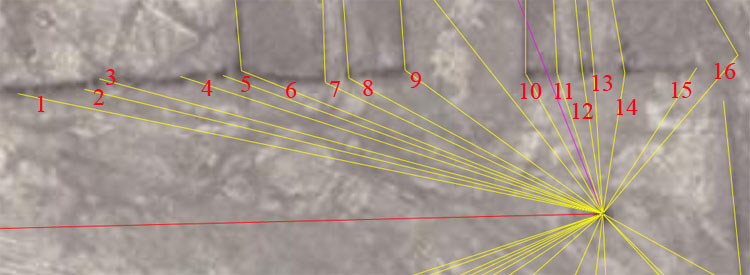A selection of coded lines are seen to run to stations above the cairn, fanning from northwest to northeast.

1 The coded distance appears to have been 453.6-feet and the angle set to 101.376-degrees.

It's probable that this position was multi-coded and over a very small range carried other scientific information and tutorials in the closely related, marked lengths and angles. Position one is given as an example of one of the centralised markers that can be seen on the site. These interim markers look a bit like little earthen bridges and it's sometimes a a bit difficult to establish the precise point of resolution.

The 453.6-feet distance would code the vertical height of the Great Pyramid to the top of its altar floor. In all recorded history the pyramid was never seen to have a capstone. A calculation matrix design on its top would have been very useful for gathering scientific information about solar, lunar, planetary or stellar rises and sets around the horizon, using a large, swiveling alidade sighting rule over the expansive plane-table altar, which measured 44-feet square.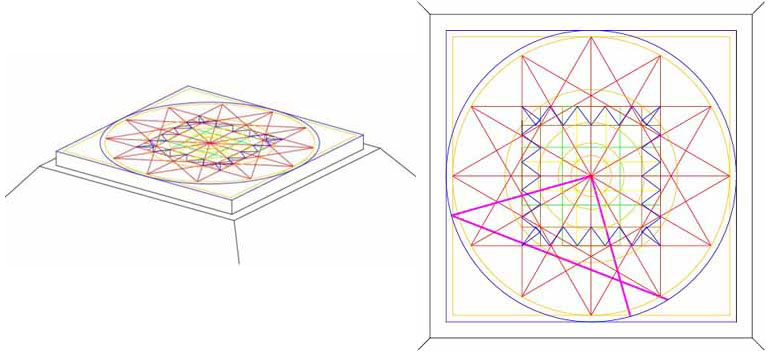Based upon the geometric overlay system of star and cross patterns used by the ancient Celts and partially preserved by surviving groups with links to the ancient "Masters of the Craft", like the Masons, the calculation matrix atop the Great Pyramid should have looked approximately like this. A very similar matrix design is found in the hill country above Nazca and another variation on the design was incorporated into the Bush Barrow Lozenge of Southern England. A series of star and cross patterns are overlaid to form a 64-square matrix and this is the origin of our chessboard. The early-epoch zodiacs of Babylon and the Middle Eastern countries would also include a 12-pointed star to indicate the 12-houses of the Zodiac, spread 30-degrees apart around the ecliptic. In the picture to the right a special "purple" 3,4,5, triangle is included. As at Stonehenge, where the 56-posts of the Aubrey Circle acted as azimuth or angle stations and utilised a swiveling 3,4,5, triangle (using measuring ropes pulled between static post stations) of this sort to determine precise angles of stellar rise and set on the embankments, so also would the Great Pyramid's altar have, conceivably, functioned in a similar way. To see how the huge swiveling 3,4,5 triangle worked at Stonehenge to provide accurate degree angle fixes onto the alighting or rising positions of stars, CLICK HERE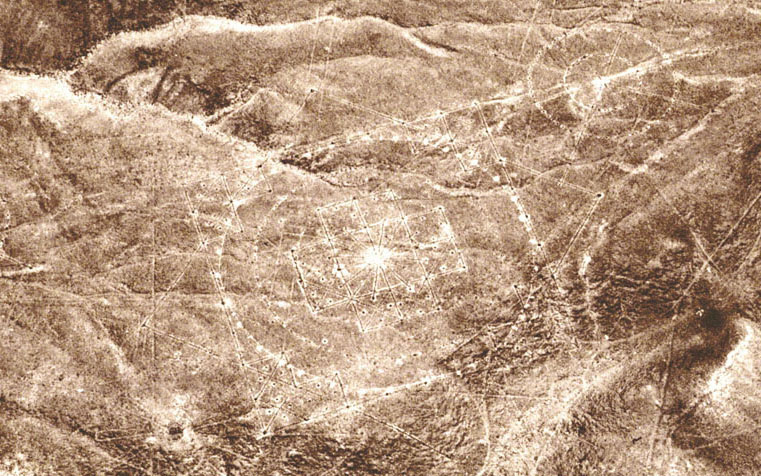The calculation matrix etched into the ground in the hill country near Nazca above the desert geometry and glyphs. A very similar matrix is found to be purpose designed into the terrain at Rennes Le Chateau in Southern France. A full explanation of how star and cross patterns geometry forms the 64-square calculation centre is explained within this website. This design pattern constitutes a kind of "Holy of Holies", sacred-geometry for ancient European nations and anyone who understands the workings of each component part of the multi-layered geometry would be sufficiently adept to set up fully functioning and potentially abundant "CIVILISATION" anywhere in the world.

These matices, found around the world, either as marked ground geometry or as zodiacal and memory-device artefacts, varied somewhat in the codes that they contained. At both Rennes Le Chateau and Stonehenge the concentric "square & circle" aspects of the geometry mostly reduced in size according to (essentially) the PHI ratio (1 to 1.6180339) for each inward layer, although "Z" Holes at Stonehenge (132-feet diameter) was straight navigational coding.
At Stonehenge there is a marker on the "Avenue" indicating the edge of a circle that has a diameter of 466. 56-feet (navigational coding), which reduces by (essentially) PHI to the diameter of the Aubrey Circle (288-feet diameter ... navigation), which reduces by PHI to the "Y" Holes Circle (178.2-feet ... navigation), which reduces by PHI to the Sarsen Circle (110-feet ... navigation).
The Nazca matrix, by consequence, contains 4 concentric circles, each coding mostly navigational numbers, but with one strong lunar code represented by the 2nd inward circle (see explanation accompanying the gyph picture below).

As stated, the return angle from position 1 to the cairn surveying station is visually set to 101.376-degrees (1013 & 19/25ths ÷ 10). The stybolite platform upon which the Parthenon of Athens sits has an intended design width of 101.376-feet to represent 1-second of arc for an earth equatorial circumference of 24883.2 British Standard Miles of 5280-feet each. Alternatively, an angle of 101.25 would also be very significant to both lunar and navigational calculations. There would be 3.5 periods of 101.25-days in a lunar year of 354.375-days and 101.25-feet would be 1/7th the design length of the Khafre Pyramid (Egypt's Pyramid of the Moon).

2. The length from the cairn to this marked station is 405-feet at an angle of 283.5-degrees.

In both distance and angle the coding is primarily lunar. In the Sabbatical Calendar count of the Moon's period of 7.2-lunar years (2551.5-days) there would be 63 intervals of 40.5-days (972-hours). In the 25920-year count for the duration of the Precession of the Equinoxes there would be 64-periods of 405-years. The perimeter value of the Khafre Pyramid (2835-feet) equates to 405-feet X 7. There would be 8.75 intervals of 40.5-days in a lunar year.

The angle of 283.5-degrees from the cairn to this station is self-explanatory. The sum of 2835-days is 8 lunar years and 2835-feet was the design perimeter value for one circumnavigation of the Khafre Pyramid. It was also a carefully marked design-length within the Octagon geometric earthmound complex of Newark Ohio.

3. The distance is 396-feet at an angle from the outer-marker station back to the cairn of 105-degrees.

The 396 value is a part of a mathematical progression that relates to the equatorial circumference of the Earth, as measured by the mile of 5280 ("11" family of numbers). The progression goes: 396, 792 (the Earth is, quite literally, 7920 British miles in diameter), etc.

The angle codes the value for the Hebrew Reed measurement of 10.5-feet (10-Greek feet of 12.6-inches each or 5 Assyrian cubits) The Great Pyramid @ 756-feet long is 72-Reeds in length. The Greek mile of 5250-feet is 500-Reeds and the Greek stadia of 630 feet is 60-Reeds. There would be 243 intervals of 10.5-days (252-hours) in the Sabbatical Calendar lunar count of 2551.5-days. There would be 243.5 intervals of 10.5-days in the Sabbatical Calendar solar count of 2556.75-days (7 solar years). There would be 270 increments of 10.5-feet in one circumnavigation of the Khafre Pyramid, etc.

4. The distance is 337.5-feet @ 108-degrees return to the cairn.

A mathematical progression based upon 337.5 is primarily lunar, but the values derived are also very useful for navigation. There would be 756 intervals of 33.75-days in the 2551.5-day (7.2 lunar year) count of the moon within the Sabbatical Calendar.

The angle in this instance (108-degrees) renders a value that was very important to navigation, the lunar period, the Precession of the Equinoxes count and the equatorial size of the Earth. The progression goes: 108, 216 (note that under the 25920-year duration of Precession the sun spends 2160-years in each house of the zodiac... the British bushel was 2160 cubic inches, the Babylonian Homer was 21600 cubic inches, the Hebrew Desert Standard Log was 21.6-cubic inches, the moon is 2160-miles in diameter), 324, 432 (the Great Pyramid is 432 Celtic cubits of 21-inches each in length), 540 (multi-purpose value of immense importance and especially for lunar counts), 648, 756 (length in feet of the Great Pyramid... 1/8th of 1-second of Earth Equatorial arc), etc.

5. The distance is 307.2-feet at an angle of 110-degrees.

The 307.2 constituted a part of a navigational mathematical progression, based on using the value 24883.2-miles (Greek or British) to describe the equatorial circumference. Amongst the values that occur using this progression is 101376. Remember that the stybolite platform of the Parthenon is 101.376 feet wide and that this represents 1-second of arc for a world of 24883.2 British standard miles of 5280-feet each. There are 3072 Egyptian Royal Cubits of 20.625-inches each in a British Standard mile.

The angle of 110-degrees is part of an 11-family progression used in navigation by the ancient British and Germanic tribes. The British distance measures into modern times were divisible by 11. This includes: League (16500-feet), Mile (5280-feet), Furlong or furrowlong (660-feet), Chain (66-feet) Rod or Perch (16.5-feet), Fathom (originally 5.5-feet, but in recent centuries modified to 6-feet) & Link (7.92-inches). If the Great Pyramid @ 756-feet were symbolically elongated 3-inches to 756.25-feet, then this would comply perfectly to the British "11" navigational system. The wonderful mathematical window of opportunity offered is that any linear sea-legs completed in 11-series increments will provide a diameter that converts to a sexagesimal circumference using PI @ 314&2/11ths ÷ 100 (3.141818182). The Greeks used PI @ 22 ÷ 7 for their system of navigation (3.142857143 ...or 3 & 1/7th).

6. The distance to this very important corner position where a secondary line commences is 295.3125-feet (295 & 5/16ths-feet) and the angle from the cairn to this position is 291.6-degrees.

The distance is, of course, coding the duration of the lunar month at 29.53125-days. This length would be 1/2 the design length on the diagonal (hypotenuse) running up the faces of the Khafre Pyramid to its apex (590.625 feet) based upon the pyramid being built to the formula of a 3.4.5-triangle. The dimensions are: (adj.) 354.375-feet...1/2 the base length, (opp. 472.5-feet)... height and (hyp.) 590.625-feet... diagonal. Sir William Flinders Petrie, in his assessment of the Khafre Pyramid, agreed it was built to the 3,4,5-triangle principle.

The angle (291.6-degrees) is both navigational and lunar in nature and also has a strong association to the Roman Pace (58.32-inches... 29.16-inches X 2) and foot (11.664-inches). The Bush Barrow Lozenge artefact of Southern England, used at Stonehenge, is 7.29-inches long or 1/8th of a Roman Pace. It dates to a time of perhaps 2000-years before there was a Roman Empire. All of the values used at Nazca can be traced back to the Pyramids of the Gizeh Plateau and Saqqara, long before the advent of Roman & Greek civilisations.

7. The distance is 233.28-feet (233.3333) at a return angle back to the cairn of 115.2-degrees.

This distance would be 240 Roman feet or 48-Paces (of course the Romans never invented the increment, only adopted it from earlier cousin civilisations at a very late era). The Roman measure introduces yet another navigational system for traversing the vast oceans. Under this system any linear diameter, using a Roman mile of 5000 Roman feet of 11.66666-inches each converts to a perfect "11" family value on the circumference of a circle, using PI @ 22/7ths. This window of mathematical opportunity could be exploited when doing positional plotting at sea to keep track of degree angles back to the point of departure or onwards to the destination. The Roman method was similar to the Greek method, whereas the British method converted an "11" family diameter into a sexagesimal circumference divisible by 360-degrees (Babylonian 360-degree method). The early cousin nations used a variety of systems to circumnavigate the world safely and set up either temporary mining operations or long term civilisations on several far-flung continents.

It's very obvious that there was considerable interaction and trade going on between Peru-Bolivia with ancient Egypt, inasmuch as there was widespread use of South American substances in Egypt ( Nicotine, Cocaine). The use of sedatives was widespread in ancient Egypt and the substances positively detected in ancient mummies also include Hashish, a Cannabis-Marijuana derivative from Asia. To see one article that shows the serious infighting in academic circles over the "unwanted find" of Nicotine & Cocaine in millennium's old Egyptians, thereby demolishing the classicist-isolationist religion, theoretical base and orthodox view concerning world history CLICK HERE

The angle in this instance is 115.2-degrees and this is a very important navigational value. The Sarsen Circle at Stonehenge was capped by 30 horizontal lying lintels, wherein the outer-rim sweep per lintel capstone averaged 11.52 feet, for a full circumference of 345.6-feet. A mathematical progression based upon 11.52 is very important to navigation. In viewing the equatorial size of the Earth as 12 X 12 X 12 X 12 X 1.2-miles or 24883.2-miles (either Greek of British), the sum of 115.2-miles would be 1/216th part. The actual surface area of the 4 faces of the Great Pyramid were 230400-square feet each (8-Egyptian acres) or 115200 X 2. Amongst its several uses, this very versatile value was also used to correct the Sothic year (365.25-days to the true duration of the year, by deducting 11.52-minutes from 365.25-days.

8. The distance is 218.75-feet at an angle of 118.125-degrees.

The distance value is a part of a mathematical progression that relates to lunar and navigational calculations. The progression goes: 218.75, 437.5, 656.25, 875 (at the end of the Sabbatical Calendar count in the Sabbatical year, there were 8.75-days of deficit error), 1093.75, 13125 (important navigational value), 1531.25, 1750 (very important navigational value... also used as a ratio (1.75) to convert seven-based fractions to whole numbers...eg... 360 ÷ 7 = 51.42857143 (X 1.75) = 90), 1968.75 (This value was used copiously in navigation. It also was used in both the Egyptian and Roman standards for weighing gold. In both instances the "Beqa" weight was 196.875-grains. The numbers found on the ground at Nazca were also the numbers used in all ancient Mediterranean and European Weights, Measures and Volumes.

The return angle back to the cairn surveying station is 118.125-degrees. This value in days represents 4 lunar months of 29.53125-days each. This value in feet represented the base value for creating the Khafre Pyramid under the geometric method of the 3,4,5 triangle. The sum of 3 X 118.125-feet (354.375-feet) represented half the base length or the "adjacent". The sum of 4 X 118.125-feet (472.5-feet) represented the vertical height or "opposite". The sum of 5 X 118.125 represented the face diagonal or "hypotenuse".

9. The distance is coded to be 185.625-feet (185 & 5/8ths) and the return angle to the cairn is 126-degrees.

The distance is part of a slightly more complex navigational progression, which is based upon the mile of 5280-feet. When the Roman Pace of 58.32 is divided by 18.5625 the result is 3.141818182, which is the PI value used in navigation to convert distances covered in miles of 5280 feet or leagues of 16500-feet (3.125-miles) into sexagesimal (divisible by 60) circumferences. Hence: 16500-feet X 3.141818182 = 51840-feet or 144-feet (12 X 12) per degree. The sum of 314.18181818 is 314 & 2/11ths.

The degree angle is an expression of the Greek foot (12.6-inches) and mile (5250-feet), as well as the Hebrew reed (10.5-feet), etc.

10. The distance to this corner position is 121.5-feet at an angle of 115.2-degrees.

The distance of 121.5-feet codes a value that was very important to the lunar cycle and navigation. The progression went: 121.5 (there would be 21 intervals of 121.5-days...which is 2916-hours... in the 2551.5-days that constituted the lunar count within the Sabbatical Calendar), 243, 364.5 (in days this lunar count was only .75 of a day off the solar year and ancient farmers were using a year count of 364-days until the "correction" festival at the end of the seventh or Sabbatical year), 486 (the half "reed" measurement was 5.25-feet and could be used as a calculator for keeping track of the positions of both the sun and the moon during the Sabbatical cycle. There were 486 intervals of 5.25-days in the 2551.5 lunar count and 487 intervals of 5.25-days in the 2556.75-day solar count. The length of the Celtic Calendar of Coligny of ancient France is 4.86-feet. The length of a Roman mile was 5000 Roman feet or 4860 British standard feet, etc.), 607.5, 729 (the length of the Bush Barrow Lozenge memory device of Southern England was 7.29-inches or 1/8th of a Roman Pace), 850.5 (navigation), 972 (navigation)...1944 (navigation), etc. This value is at the basis of one of the most important mathematical progressions of antiquity

The return angle to the cairn is 151.2-degrees. The Great Pyramid has a side length of 756-feet and the total for two sides is 1512-feet or 1/4th of one minute of Earth equatorial arc. The value relates primarily to navigation, but was also used for tracking the lunar period within the 6804-day lunar nutation cycle (major standstill to minor standstill and back to major standstill). There were 45 interval of 151.2-days (3628.8-hours) in the 6804-day cycle. It's worthy to note that one circumnavigation of the Great Pyramid (3024-feet) was 36288-inches for 1/2 a minute of Earth equatorial arc and that there were 362880-feet in 1-degree of arc according to the Great Pyramid's geodetic system. Under certain ancient mathematical systems in place, one finds the brilliant use of the same values to describe both lunar periods and principles of navigation simultaneously. The Greek system, supposedly acquired by them from the Egyptians, demonstrates this capacity perfectly.

11. The distance is 112-feet at a return angle to the cairn of 162-degrees.

The value 112 was very important within the Great Pyramid measurements and 27 X 112-feet would represent one circumnavigation of the Great Pyramid. It was a part of a mathematical progression that related to navigation. The Great Pyramid's value for 1-second of equatorial arc would be 100.8 feet and 11.2-feet would be 1/9th of that value. If the full equatorial circumference is given in feet then the Great Pyramid's value is 24883.2 X 5250 (Greek mile) = 130636800 British feet. This value divided by 1120 = 116640. It will be recalled that the Roman foot was 11.664-inches. If the 130636800-feet value was converted to Greek feet of 12.6-inches, then there would be 12441600 such feet in the equatorial circumference.The sum of 24883.2 is 124416 X 2. If the 130636800-feet value was converted to the larger of the Egyptian Royal Cubits (1.728-feet or 20.736-inches) then there would be 75600000 in the circumference of the Earth. If the 130636800-feet value was converted to Roman feet of 11.664-nches, there would be 134400000, etc. The known ancient measurement standards of all the cousin nations will dovetail into each other like this and are merely different ratio expressions of the selfsame mathematical parcel shared by all. With regards to the larger Egyptian Royal Cubit @ 1.728-feet, it is interesting to note that a British cubic foot (12 X 12 X 12-inches) = 1728 cubic inches.

An early Egyptian weights system was made up of the "Kite", "Deben" and "Sep". When King Henry VIII reformed the British weights system he essentially adopted this early Egyptian standard to replace yet another ancient standard in general use in England. The new standard was made up of of an ounce (10 Deben or 100 Kite) and 16 such ounces made 1 British pound. The sum of 112-pounds made 1-cwt (hundredweight) and 20 such cwt made 1-ton (2240-lbs). The former British measurement, volume and weight systems, that have recently been replaced by "metrickery", have roots in ancient Egypt from before 5000 BC.

The degree angle from this outer-marker station to the cairn is 162-degrees. This is coding "rounded PHI" @ 1.62 or pure PHI @ 1.6180339 (see explanation on PHI coding within the dimensions and angles of the Great Pyramid... above).

12. The distance is 108.864-feet (1088 & 13/20ths ÷ 10) and the return angle to the surveying cairn station is 171.875-degrees (171 & 7/8ths).

The distance codes a very important value in a navigational progression, which increases to the value 1306.368 in 12 steps. This is, of course, related to the Great Pyramid's standard for the equatorial circumference of the Earth (130636800-feet) and this length at Nazca would be 1/1200000th of the equatorial circumference. The value was also marginally exploitable for measuring intervals within the lunar nutation cycle of 6804-days. The position would have been dual coded to also mean 108-feet, a very important navigational increment.

The angle is 171.875-degrees and this was a very important value that related to the equatorial circumference of the Earth under the "11" system, with a mile of 5280-feet. Depending on the navigational system being used, the Egyptians had slightly different "Royal Cubits" of length. These were: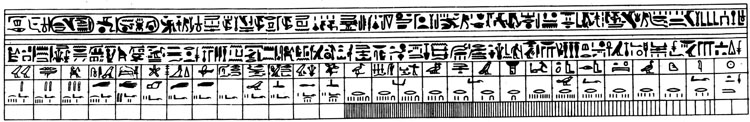• The formula used to create the most well-known Egyptian Royal Cubit was: 24883.2-miles of 5250-feet each = 24741.81818- miles of 5280-feet ... ÷ 1200 = 20.61818182. This is the published length of the "Royal Cubit" and an exemplar of this size is housed within the Turin Museum. This size also complies to the Great Pyramid's length, which was 440 Egyptian Royal Cubits. Therefore 9072-inches (756-feet) ÷ 440 = 20.61818182-inches. In other words, using the Egyptian Royal Cubit symbolically to represent "miles" of 5280 feet, this particular cubit represents 1/1200th of the Earth's equatorial circumference under the Great Pyramid's literal base length and height.

• If the Great Pyramid was elongated by 3-inches, then the reading per side would be 756.25-feet and this system would make the Great Pyramid comply to the ancient British-Celtic system of navigation under the "11" family of numbers. The inch value for the pyramid's side length would be 9075 and this value ÷ 440 = 20.625-inches. If this cubit was symbolically read as miles, then 1200 of them would give an Earth equatorial circumference of 24750-miles. This value and navigational system was the preferred one adopted and used by many early European mariners and is positively represented at Nazca. There are 3072 Egyptian Royal Cubits of 20.625-inches each in the British Standard mile of 5280-feet.

• Amongst the largest of the Egyptian Royal Cubits is one of 20.736-inches and this one was very special. Whereas viewing the Earth as 24741.81818-miles (literal Great Pyramid standard) was excellent for navigation using the "6&7" family of numbers, it did not scientifically describe the true size of the Earth. It only provided a very "near" value that was fluidly factorable down to as little as 1-inch or fractions thereof. This close proximity value of the Earth's true dimensions was more than sufficient to ensure safe traversals of the great oceans, using an easily manipulated mathematical system for accurate positional plotting. The British "11" system described the size of the Earth with slightly better accuracy, but was also based upon factored values down to 1-inch or fractions thereof. The most accurate factorable system described the Earth as 12 X 12 X 12 X 12 X 1.2-miles of 5280 feet or 24883.2 British miles (131383296-feet) This value in miles (24883.2) ÷ 1200 = 20.736*.

Sir William Flinders Petrie measured the coffer in the Great Chamber of the Khafre Pyramid and accurately determined it had an outer length of 103.68-inches. This value doubled = 207.36-inches (207 & 9/25ths). In other words, the coffer was 5 cubits long using a cubit of 20.736-inches or 1.728-feet. The same cubit was represented in the dimensions of paving slabs at the base of the Great Pyramid. One of several codes carried in the length value of the coffer was the speed at which the Earth rotates in miles per hour. Therefore: 24883.2-miles ÷ 24-hours = 1036.8 MPH. Interestingly enough, the "Stirling Jug" of Scotland had a capacity of 103.68 cubic inches and this paralleled the Jerusalem Liquid Standard of the ancient Hebrews (no relationship to the present-day people calling themselves Jewish or Jews), wherein a "Cab" was 103.68-cubic inches. The value of 24883.2-miles to describe the Earth's equatorial size is in deficit error of only 18.8-miles.

• Each of the Egyptian Royal Cubits, beside coding equatorial circumference of the Earth measurements and methods according to three separate systems, also coded the speed at which the Earth rotates. To get the speed of rotation under each system, simply halve the inch value of the cubit under consideration and multiply by 100, then read the value acquired as MPH. The true rotational speed is very close to 1037.583333 MPH (1037 & 7/12ths), so a reading of 1036.8 MPH is only off by .783333 MPH.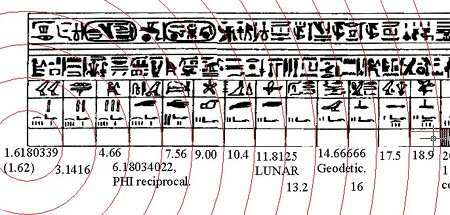The segments of an Egyptian Royal Cubit of 20.618-inches in length (as displayed at the Turin museum). The 1st interval is a PHI inch (1-inch X 1.6180339); The 2nd interval is a PI-inch (1-inch X 3.1416); The 3rd interval seems to be coded at 4.6656-inches (navigational progression); The 4th interval is a special PHI reciprocal value that was absolutely essential for the fabrication of correct (precise capacity) round volume vessels for the ancient market places... for example: the ancient British Bushel was a round tub with a base diameter of 3 X 6.18034-inches and straight sides above the base 8-inches high, giving an exact capacity of 2160-cubic inches); The 5th interval is navigational (note: the Great Pyramid is 756-feet long), etc.
The Royal Cubit layout seems to have been unsuitable as a rule used in building work, but functioned more as a specialised calculator and memory device. Other, more factorable, cubit or foot rules would have been used in construction work.

The return angle of 171.875-degrees to the cairn is in deference to the British-Celtic navigational system and the value of 1.71875-feet would be 20.625-inches.

As stated, the Nazca geometry was set out for the purpose of teaching initiates how to become navigators and astronomers and after several years of grueling training in this open air university the lessons would have been indelibly fixed in the mind of the student, ...so intense was the course of instruction.

13. The length is 108-feet and the angle from the cairn to this position is 356.4 degrees.

The length value was part of a mathematical progression that produced numbers useful in navigation, Precession of the Equinoxes calculations, the lunar period and the Sabbatical Calendar system.

The angle relates to navigation. Its half value, 178.2 (in feet) is the width of the "Y" Holes circle at Stonehenge. The value also features prominently in a PHI reduction of the circles at Stonehenge wherein the Aubrey Circle (288-feet) ÷ PHI (1.6180339) = the diameter of the "Y" Holes Circle (178.2) ÷ 1.6180339 = the diameter of the Sarsen Circle (110-feet).

The value is a part of the "11" family of numbers related to the equatorial size of the Earth in miles of 5280 feet. It is a value that occurs in a mathematical progression that goes: 39.6, 79.2, 118.8, 158.4, 198, 237.6, 277.2, 316.8, 356.4....950.4....990....1306.8....1900.8 (the Roman Amphora was 1900.8 cubic inches)....28512, etc. Some chosen values from this progression were incorporated into the ancient Hebrew Sepphoris Dry Volume standard, wherein a Log was 3.96 cubic inches, a Cab was 158.4 cubic inches, a Seah was 950.4 cubic inches, an Ephah was 2851.20 cubic inches and a Homer was 28512 cubic inches.

A very probable explanation for this value is that it represented a substitute value for 360-degrees in a compass when navigating at sea using the Greek "6&7" method. This is how it worked:

• At sea a sailing ship is at the mercy of the wind and has to zigzag or tack on the wind in order to make its erratic way to a destination. This means legs of travel in the general direction, while crossing back and forth over the direct line to the target port. In any tack the navigator would allow the direction of travel to endure for only so long and when the ship was getting too far off the central lay line to the destination would call to the helmsman to head back towards the true line.

• Each straight line distance covered in a leg of travel has to be recorded, so that the navigator can stay abreast of where the ship sits with the vast, featureless ocean. This is where the observational experience of the navigator became so imperative, as he was doing constant mental arithmetic in estimating ship speed and angle. When he was satisfied that the ship had completed a known number of leagues or miles, he would call to the helmsman, steering the ship, to start a new leg on a new, carefully chosen degree angle.

• At the completion of each leg the navigator had to accurately plot the position where the ship sat in the vast ocean. Let us say, for example, that the ship had just completed a leg of 15 Greek miles or covered a distance of 78750-feet (75000 Greek feet). The navigator now has to convert this diameter into a circle on the plotting chart and estimate anew the angle back to the point of departure, as well as the angle onward to the point of destination. For this he needs a circle that is precisely "sexagesimal" and perfectly divisible by 360-degrees.

• The navigator puts his circle drawing compass point at the exact centre of the line and draws a perfect circle that touches each end of the line. He now uses the formula for finding PI... 22 ÷ 7 = 3.142857143 (3 & 1/7th) and multiplies the length of the last leg of travel (in feet of 12-inches) by this value. This means 5250 feet X 15 = 78750-feet X PI = 24750-feet of circumference for the new circle.

• It will be observed that the the circumference value is perfectly divisible by "11", in fact the circumference is exactly 15 British leagues of 16500-feet each, as a Greek mile converts to a British league (3.125 British miles) using PI @ 22/7ths.

• So, instead of having the circumference set to 360 calibration marks to designate each degree, why not opt for 396 marks or 360 X 1.1. This means that every degree would be based upon a value divisible by 11.

• Alternatively, a circuit of 356.4 is 3.6 less than 360 or .99 X 360.

• In this case the leg (diameter) produced a circumference of 247500-feet or 687.5 feet (125 ancient fathoms of 5.5-feet each) per degree or 625-feet X 1.1 per degree.

The essential element is that with an "11" based rule, accurate degree angles could be easily calculated and a mathematical system for precise positional plotting existed. It is interesting to note that the ancient German artefact, The Sky Disk of Nebra, is 1-Greek foot wide (12.6-inches), which converts to an outer ring circumference (using PI @ 22/7ths) of 39.6-inches or 360 X .11-inches. The Sky Disk even had 39-holes around the outer rim (to infer 39.6, in keeping with the circumference calculation based upon a diameter of 12.6-inches). The Vikings are known to have used a rule of 11-inches and the Saxon foot was 12 X 1.1-inches (13.2-inches) Many other ancient Scandinavian measurements comply to increments within the Greek (6&7) system for linear travel converting to an 11-based circumference.

14. Again, this distance appears to be 108-feet and the return angle to the cairn is 189-degrees.

The 108-feet distance is a dynamic navigational number, whereas the degree angle codes an increment of length within the base length of the Great Pyramid (756-feet long = 189-feet X 4).

The value 189 was very important within calculations of the ancient European cousin nations. The sum of 189-feet equated to 1/32nd of 1-minute of equatorial circumference arc under the Great Pyramid's literal system. The sum of 1890-days was a very important value within the lunisolar Calendar of Coligny of Celtic France. The large brass plaque offered either 64 full squares, 128 half squares alternating between 14 & 15-days, 60 named months in two parts and two CIALLOS or optional calculation sections for use as required. One had the option of using the Ciallos regions when calculating the duration of the 6804-day lunar cycle or for doing individual, customised counts (of various kinds) for the sun and moon. Otherwise, the repeats of 60 named months could unravel endlessly and the positions of both sun and moon within the Sabbatical Calendar tracked day-by-day. This, in part, is how it worked:

• To work in solar months of 30-days duration each, one used the 60 named months plus 1 intercalary Ciallos month of 30-days each, which equated to 63 X 30-days = 1890-days.
• To calculate, exclusively, the duration of the lunar month over the same panel one used all 64 squares. The way this worked was 1890-day ÷ 64 = 29.53125-days (29 & 17/32nds), which is the lunar month to an accuracy of under 1-minute.
• The sum of 3.6 periods of 1890-days or 360 X 18.9-days = 6804-days, which is the full duration of the lunar nutation cycle (major standstill to major standstill).
• The sum of 135 periods of 18.9-days was 2551.5-days, the 7.2-lunar year duration monitored alongside the 7-solar years (2556.75-days) of the lunisolar Sabbatical Calendar system.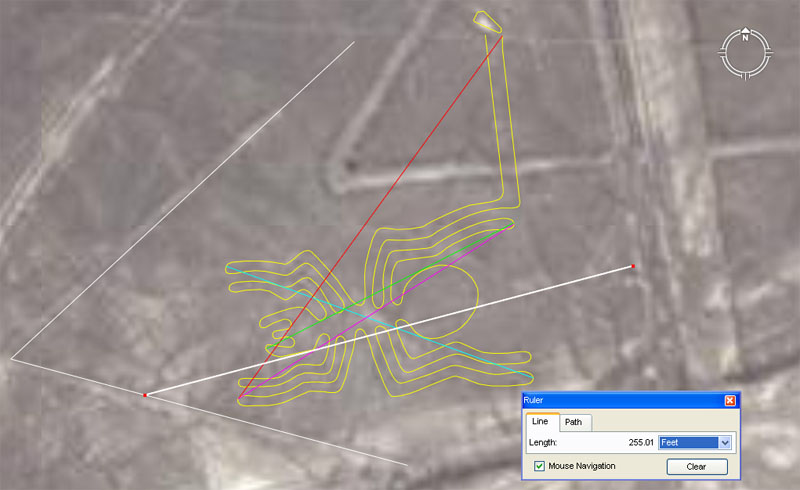The Spider at Nazca moves along a line that is 255.15-feet long and which is set to the azimuth angle of 255.15-degrees* around from north. The white line shown covers a marked ground line at Nazca, indicating the orientation and path of travel for the spider. The coding is lunar and refers to the 2551.5-days (7.2-lunar years @ 354.375-days each) counted within the ancient 7-solar year Sabbatical calendar system. The long leg of the spider sits on an azimuth angle very close to 172.8-degrees around from north and the angle code is navigational. Here is a sampling of other tutorials associated with the spider:

• A second ground marked line goes up to the left on a diagonal. It has a length of 207.36-feet (navigation, Egyptian Royal Cubit) and lies at an angle 0f 105-degrees (navigation, Hebrew Reed).
• Another ground marked line, branching off the line just mentioned, diagonals up to the right for 236.25-feet (lunar code) at an angle of 47.25-degrees (lunar code). Note: the sum of 236.25 feet is half of 472.5-feet, which is the vertical height of the Khafre Pyramid under the 3,4,5 triangle method by which it was built. The length of 47.25-feet is 1/16th of the length of the Great Pyramid (756-feet) or 1/15th the length of the Khafre Pyramid (708.75-feet).
• A red line runs for 226.8-feet (lunar code) from the spider's right rear heel to the front left paw at an angle of 216-degrees (lunar, Precession of the Equinoxes).
• A green line runs diagonally across the body for 140-feet (navigation, lunar, Sabbatical Calendar) at and angle of 243-degrees (lunar) and brushes the right side of the abdomen en route, before terminating on the left jaw or palp.
• A light blue line runs diagonally across the body from the rear outer left paw to the front right outer paw, brushing the abdomen en route. The distance is 165-feet (navigation by the "11" number family) and the return angle is 110-degrees (navigation). The distance would be 30 ancient fathoms of 5.5-feet each or 10 Rods of 16.5-feet each. These "11" family measurements survived in ancient Britain and Saxon-Germanic countries.
• A magenta-purple line runs diagonally across the body from the front left paw to the second inwards right rear paw for 165-feet (navigation) at an angle of 57.6-degrees (navigation).

These sample examples show how the glyphs were carefully fabricated to a mathematically conceived design plan that could be used in practical tutorials. Generations of initiate navigators and astronomers would have, over time, been assigned the task of deciphering the many, in-built, coded length & angle aspects of the spider. This tedious task would have been accomplished by making careful measurements and calculating precise angles, then recording all of the extracted codes for later redrafting. Undoubtedly, a small, scaled representation of the spider would then have been drawn on slate or some other markable surface by the students for close scrutiny by the tutor, who could then judge how well lessons had been learnt and principles understood.

Footnote: All of the special numbers encoded by lengths and angles at Nazca and other "open air universities" were also incorporated into the "standards" of length, volume and weight within individual European nations of antiquity. Even within a nation itself, there could be many different expressions of the "mile" from province to province, but all were in a direct ratio relationship to each other. It seems obvious that, at some ancient era, each province was assigned (by Druidic priests?) the responsibility to preserve particular numerical values found within the overall parcel of scientific numbers. Some provinces would be preserving "lunar" information, whereas others would be preserving navigational values directly proportional to the equatorial size of the Earth. Here's an example of early Germanic lengths, based upon the Flemish system, that were in use in the 13th century AD and prior. The values given in metres are taken from "official" or expert literature and show only very small drift off the original, precise increments of more remote antiquity.

ALT KULMISCHE MASZ

1 Meile = 1800 Ruten ... 7777.24 Metres (this equals 25515-feet to a tolerance of 10.5-inches in 4.86 Greek miles of 5250-feet each. It is very obvious that this ancient Germanic/ Flemish/ Prussian system was fully founded on, what's come to be known as, the Greek-mile system).

1 Rute = 15 Schuh ... 4.3218 Metres (the Rute, which was in direct ratio to the Meile, was 14.175-feet. The Khafre Pyramid of Egypt, which was a "pyramid of the moon" accentuating the lunar cycle periods, was 2835-feet in perimeter value or 200 Ruten).

1 Schuh = 0.28812 Metre (The Schuh, of which 15 made up 1-Rute, was .945-feet or 11.34-inches). This was the increment of length that Greek Historian Herodotus was told about by Egyptian priests, who stated that the Great Pyramid was 800-feet long ... Therefore: 756-feet ÷ 800 = 11.34-inches. By using a rule of this length, the Great Pyramid base perimeter could give lunar cycle readings. Three side lengths of the Great Pyramid @ 756-feet per side = 2268-feet or 1134-feet X 2. The Great Pyramid is 3200-Schuh in perimeter and the Khafre Pyramid is 3000-Schuh.

1 Elle = 2 Schuh 0.57624 Metre (The Elle was 22.68-inches and there would be 1200-Elle in three base side lengths of the Great Pyramid). Alternatively, the width of the Station Stone's Rectangle at Stonehenge was 113.4-feet or 60 Elle).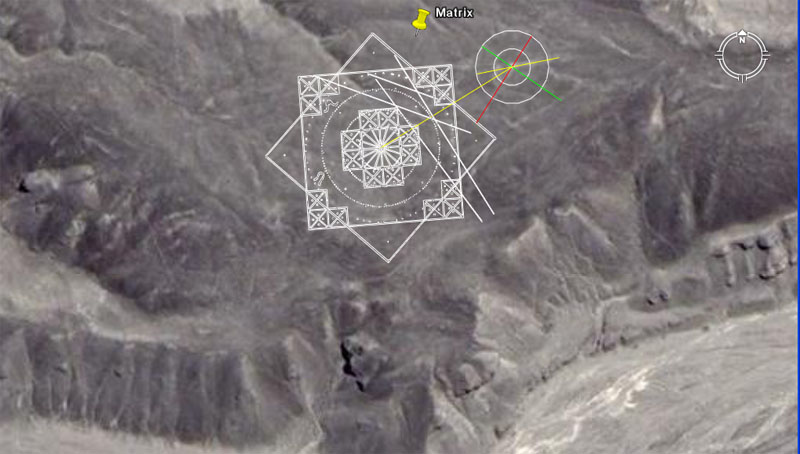The elaborate calculation matrix in the Nazca hill country lies on a azimuth angle off north of 176-degrees (coding navigation by the 5280-feet mile).

• The width of the matrix square, in one cross measure, appears to be 184.32-feet (navigation according to the equatorial circumference of 24883.2-miles .... 12 X 12 X 12 X 12 X 1.2).
• The diameter of the largest internal circle, which brushes the inner section of the perimeter square, appears to be 178.2-feet (navigation ... this is also the diameter of "Y" Holes Circle at Stonehenge.
• The diameter of the 2nd inward concentric circle appears to be 141.75-feet (lunar code); This length would be 1/5th of the length of the Khafre Pyramid.
• The diameter of the 3rd inward concentric circle appears to be 92.16-feet (navigational coding).
• The diameter of the 4th inward concentric circle appears to be 51.84-feet (navigational; used as an incremental value in calculating the duration of the Precession of the Equinoxes; the slope angle of the Great Pyramid (51.84-degrees).
• The diagonal yellow line, running to the other exterior circle appears to be 184.32-feet (navigational). It runs on an angle of 58.32-degrees (navigation and the Roman Pace measurement @ 58.32-inches).
• The outer circle of this second site appears to be 88-feet in diameter (navigation by the 5280-feet mile).
• The inwards concentric circle appears to be 44-feet in diameter (navigation by the 5280-feet mile). The diameter of 42-feet would also have been represented to teach navigation by the 5250-feet (Greek) mile.
• The yellow line dissecting this site lies on an angle of 78.75-degrees (navigation by the Greek mile of 5250-feet and an important value in calculating the duration of several lunar cycles). The length of the line appears to approximate 101.25-feet (lunar coding).
• The green line appears to run for 115.2-feet (navigation) at an angle of 124.416-degrees (coding the equatorial circumference of the Earth).
• The red line appears to run for 124.416-feet (navigation) at an angle of 32.4-degrees (navigation; Precession of the Equinoxes).

15. The distance is 132-feet and the angle is 32.8125-degrees (32 & 13/16ths).

The 132 value is a part of a very important mathematical progression to do with the mile of 5280-feet and navigation by that standard. The progression goes: 132, 264, 396, 528 (1/10th of a mile in feet), 660 (1-furlong in feet), 792 (the Earth is literally 7920-miles in equatorial diameter), etc.

The ancient Celts are known to have had a "Saxon foot" of 13.2-inches or 12 X 1.1-inches. At Stonehenge, the "Z"-Holes circle is 132-feet in diameter (which is 76.8 Egyptian Royal Cubits of 20.625-inches).

The degree angle is part of a progression that relates to the cycle of the moon and also to navigation by the mile of 5250-feet. The progression goes: 32.8125, 65.625, 98.4375, 131.25 (much used lunar and navigational increment), ... 196.875 (the Egyptian and Roman gold standards for their "Beqa" weight were 196.875-grains, ...295.3125 (in days this would be 10 lunar months), etc. Remember, that all the decimal values seen above are only simple fractions, easily calculated on a manual abacus.

16. The length through the corner position to where the secondary line begins is 158.4-feet at an angle of 40.5-degrees from the cairn.

The value of 158.4 is part of an "11" family mathematical progression that relates to the mile of 5280-feet.

The angle of 40.5-degrees relates to a predominantly lunar mathematical progression. There would be 63 intervals (9 X 7) of 40.5 days in the 2551.5-day lunar duration monitored within the lunisolar Sabbatical Calendar system. There would be 168-intervals (24 X 7) of 40.5-days in the 6804-day lunar nutation cycle. There would be 640 intervals of 40.5-years in the 25920-year period of the Precession of the Equinoxes.

Continue